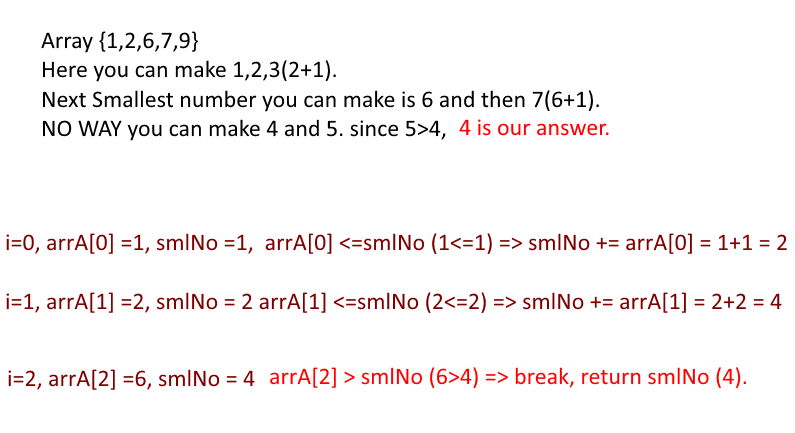Beginner

# Minimum number that cannot be formed by any subset of an array

Objective: Given a sorted array of positive integers, find out the smallest integer which cannot be represented as the sum of any subset of the array

Input: A Sorted Array

Output: The smallest number which cannot be represented as the sum of any subset of the given array

Examples :

```Array {1,1,3,4,6,7,9} smallest Number : 32
Array {1,1,1,1,1} smallest Number : 6
Array {2,3,6,7} smallest Number : 1
Array {1,2,6,7,9} smallest Number : 4
```

Approach:

• If 1 is not present in the array, our answer is 1.
• So take a variable "smlNumber" and assign 1 to it.
• Now we need to find the gap between the array elements which cannot be represented as sum of any subset of array.
• To find that keep adding the array elements to smlNumber and check it current array element and if at any point smlNumber<current array element that means we have found the gap. return smlNumber.
• See figureThe smallest number which cannot be represented as the sum of any subset of the given array

Code:

Output:

```Smallest Positive Integer that cant be represented by the sum of any subset of following arrays are :

{1,1,3,4,6,7,9} - 32
{1,1,1,1,1} -> 6
{2,3,6,7} -> 1
{1,2,6,7,9} -> 4```